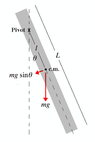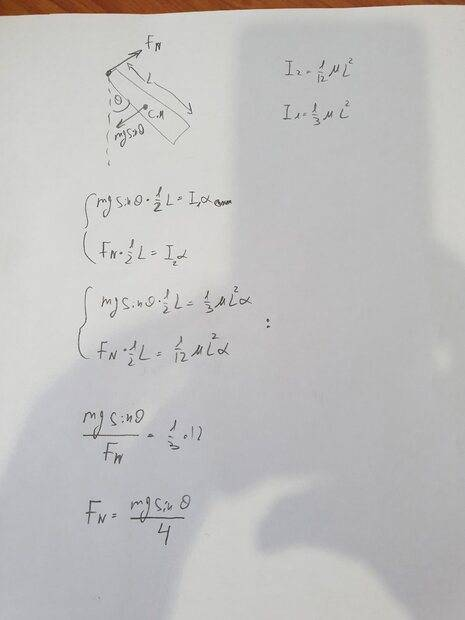# Forces that act on a physical pendulum

• Yossi33
In summary, the conversation discusses the forces that act on a rod at its pivot point as it rotates about the pivot. The question is to find the angular velocity, angular acceleration, and forces at the pivot point when the rod reaches a certain angle. The conversation delves into the concept of torque and the relationship between the gravitational force and the component of the pivot force normal to the rod. It is concluded that there are two forces at the pivot point, and the resolution of these forces is necessary to fully understand the dynamics of the rod's rotation.

#### Yossi33

Homework Statement
physical pendulum
Relevant Equations
torque=Ia
hello, i have a question about the forces that act on a rod at it's pivot point. the rod is free to rotate about the pivot point at the edge and it starts from rest parallel to the ground.the question is : when it reach to angle theta find the a] the angular velocity b] angular acceleration c] the forces acts on the rod at the pivot point (normal)
the rod is of length L and mass M
i found w^2=(3gcos(theta))/L
angular acceleration=alpha=(3gsin(theta))/2L
c- i try to calculate the normal force that acts toward the pivot point
N-mgcos(theta)=Mw^2R
and R=L/2. but it's not the answer and i searched at the interent and find that there are 2 forces act at the pivot point of the rod and i didn't understand why. thanks for the help.

#### Attachments

•images.png
2.9 KB · Views: 51
Yossi33 said:
Homework Statement:: physical pendulum
Relevant Equations:: torque=Ia

there are 2 forces act at the pivot point of the rod and i didn't understand why
If the force from the pivot were only along the line of the rod then both forces (that and gravity) would have lines of action through the rod’s mass centre. So there would be no torque to create the angular acceleration.

so what are the forces that act there, what are their direction?
and mgsin(theta) is a component of the gravity that perpendicular to the radius of rotation this force does the torque , no?

Yossi33 said:
so what are the forces that act there, what are their direction?
and mgsin(theta) is a component of the gravity that perpendicular to the radius of rotation this force does the torque , no?
The tangential component of gravity exerts a torque about the pivot, but not about the mass centre.
You can express the angular acceleration of the rod in terms of that torque about the pivot, or by the torque the forces at the pivot exert about the mass centre. Since the angular acceleration is the same either way, you can find the relationship between the gravitational force and the component of the pivot force normal to the rod.

i think i understood what you meant and tried to get the relation in this photoYossi33 said:
i think i understood what you meant and tried to get the relation in this photoView attachment 295316
Well done.

thanks man,please if you can still help me to fully understand it, because i don't understand why there has to be 2 forces .
can i treat it as a normal force that opposite the wiegh components?
and because there is a torque in the tangential axis , i can't calculate the forces only with Newtons second law?
mgsin(theta)-Fn(tangential)=ma(tangential)
or this is another way
?

Last edited:
Yossi33 said:
thanks man,please if you can still help me to fully understand it, because i don't understand why there has to be 2 forces .
can i treat it as a normal force that opposite the wiegh components?
It's not that there are two forces. The reaction from the pivot is one force, but you can opt to resolve it into two perpendicular forces in any way you choose.
If you resolve it into one component along the rod and one normal to it then both are nonzero.
Yossi33 said:
can i treat it as a normal force that opposite the wiegh components?
Not sure what you mean.
Yossi33 said:
because there is a torque in the tangential axis , i can't calculate the forces only with Newtons second law?
Torques have no relevance to ##\Sigma F=ma##.
Yossi33 said:
mgsin(theta)-Fn(tangential)=ma(tangential)
That's correct, if by Fn(tangential) you mean the component normal to the rod of the reaction force at the pivot.